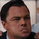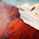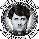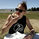81221 vizualizações
MACD – Moving Average Convergence Divergence. The MACD is calculated
by subtracting a 26-day moving average of a security's price from a
12-day moving average of its price. The result is an indicator that
oscillates above and below zero. When the MACD is above zero, it means
the 12-day moving average is higher than the 26-day moving average.
This is bullish as it shows that current expectations (i.e., the 12-day
moving average) are more bullish than previous expectations (i.e., the
26-day average). This implies a bullish , or upward, shift in the supply/demand
lines. When the MACD falls below zero, it means that the 12-day moving average
is less than the 26-day moving average, implying a bearish shift in the
supply/demand lines.
A 9-day moving average of the MACD (not of the security's price) is usually
plotted on top of the MACD indicator. This line is referred to as the "signal"
line. The signal line anticipates the convergence of the two moving averages
(i.e., the movement of the MACD toward the zero line).
Let's consider the rational behind this technique. The MACD is the difference
between two moving averages of price. When the shorter-term moving average rises
above the longer-term moving average (i.e., the MACD rises above zero), it means
that investor expectations are becoming more bullish (i.e., there has been an
upward shift in the supply/demand lines). By plotting a 9-day moving average of
the MACD , we can see the changing of expectations (i.e., the shifting of the
supply/demand lines) as they occur.
Script de código aberto

No verdadeiro espírito TradingView, o autor deste script o publicou com código aberto, para que os traders possam compreendê-lo e verificá-lo. Um brinde ao autor! Você pode usá-lo gratuitamente, mas a reutilização deste código em uma publicação é regida pelas Regras da Casa. Você pode favoritá-lo para utilizá-lo em um gráfico.

Quer usar esse script no gráfico?
```////////////////////////////////////////////////////////////
//  Copyright by HPotter v1.0 18/06/2014
// MACD – Moving Average Convergence Divergence. The MACD is calculated
// by subtracting a 26-day moving average of a security's price from a
// 12-day moving average of its price. The result is an indicator that
// oscillates above and below zero. When the MACD is above zero, it means
// the 12-day moving average is higher than the 26-day moving average.
// This is bullish as it shows that current expectations (i.e., the 12-day
// moving average) are more bullish than previous expectations (i.e., the
// 26-day average). This implies a bullish, or upward, shift in the supply/demand
// lines. When the MACD falls below zero, it means that the 12-day moving average
// is less than the 26-day moving average, implying a bearish shift in the
// supply/demand lines.
// A 9-day moving average of the MACD (not of the security's price) is usually
// plotted on top of the MACD indicator. This line is referred to as the "signal"
// line. The signal line anticipates the convergence of the two moving averages
// (i.e., the movement of the MACD toward the zero line).
// Let's consider the rational behind this technique. The MACD is the difference
// between two moving averages of price. When the shorter-term moving average rises
// above the longer-term moving average (i.e., the MACD rises above zero), it means
// that investor expectations are becoming more bullish (i.e., there has been an
// upward shift in the supply/demand lines). By plotting a 9-day moving average of
// the MACD, we can see the changing of expectations (i.e., the shifting of the
// supply/demand lines) as they occur.
////////////////////////////////////////////////////////////
study(title="MACD Crossover", shorttitle="MACD Crossover")
fastLength = input(8, minval=1)
slowLength = input(16,minval=1)
signalLength=input(11,minval=1)
hline(0, color=purple, linestyle=dashed)
fastMA = ema(close, fastLength)
slowMA = ema(close, slowLength)
macd = fastMA - slowMA
signal = sma(macd, signalLength)
pos = iff(signal < macd , 1,
iff(signal > macd, -1, nz(pos, 0)))
barcolor(pos == -1 ? red: pos == 1 ? green : blue)
plot(signal, color=red, title="SIGNAL")
plot(macd, color=blue, title="MACD")
```
Donate BTC: 13fXLkhWuGMXRmcvwkG2gaWKcnsiD88bwE
USDT (TRC20): TH29EEXa19vfwZNYvxdUuMxoFY5QDYLcWG

## Comentáriosmizteranderson
@mizteranderson, use the function cross() to detect crossings, but even better crossover() will only detect if its higher than the 2nd value.
macd_cross = crossover(macd,signal)
Respostaabhishektomar1796
@abhishektomar1796, I dont think there is a lot of difference... Maybe a slight different Moving Average but maybe the Author can let us know @sketchytraida
RespostaThis indicator seems pretty good! I would just have a question. Is it possible to set an aler when the "MacdSE -2 or +2" appears? I am not too sure what it means either :)

thanks!
RespostaConfused as to why the input lengths differ from the description (8,16,11 compared to the standard 12,26,9). The result seems so react quicker to movements but... why? Thanks :)
Resposta